# Do Newton's laws of motion have restrictions

### 1.3.3 Newton's Laws (modern formulation)

After we have defined kinematic quantities for the movement of a mass point and scaled them using a suitable system of measurements, we can now formulate Newton's laws
(1)
A body is at rest or moves at a constant speed when there is no force acting on it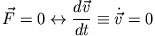(2)
The change in momentum over time (the momentum is) of a body is equal to the force acting on the body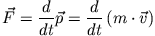(3)
Two bodies exert the same amount of forces on each other, but oppositely directed forces (actio equals reaction). Is called with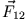the force from mass 2 to mass 1 and with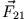the opposite situation applies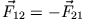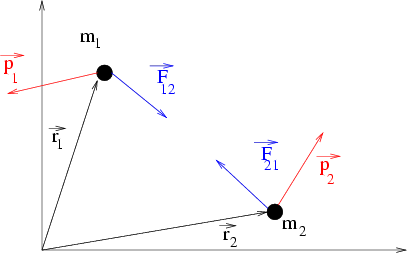These three laws are sufficient to understand classical mechanics: they describe motion processes on earth, in the solar system or the dynamics of galaxies. However, we will formulate restrictive conditions later. Put simply, the restrictions are: Newton's laws apply to systems that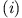move much slower than light in a vacuum and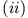are macroscopic.

Remarks:

(i)
Newton's synthesis consists in the abstraction that the laws for the movement of bodies on earth are the same as in space.

(ii)
The first law says that constant speed movement is the natural state of a force-free body. Since we cannot in principle define an absolute reference system, the first law allows us to choose any system that moves with constant speed - inertial system: every inertial system is equivalent to the description of physical processes (principle of relativity).

(iii)
From the second law it follows for masses that are constant in time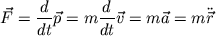There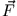usually from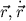and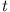we have an equation of motion for a classical system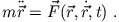This is an ordinary 2nd order differential equation for determining location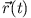and impulse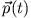of a system. We need to know the forces at work and the initial state of the system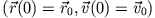, everything else is clearly determined by the equation of motion.

(iv)
A consequence of the third law is the conservation of momentum in a closed system of mass points. This is understood to mean a system on which no external forces act. For two mass points, the sum of their momentum applies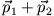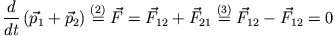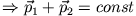The corresponding extension for N mass points is analogous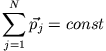The total momentum of a system of mass points is the same at all times and is therefore a conservation quantity if only internal forces between the mass points and no external forces act on the system.

(v)
So what is a force? Mechanics don't give us an answer to that.
How do we experience a force? E.g. in the form of Newton's law of gravitation (details later)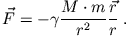It applies to the falling apple (M: mass of the earth, m: mass of the apple) as well as to the movement of the planets (M: mass of the sun, m: e.g. mass of the earth).
What does the force do? According to the second law, it causes a change in momentum and thus an accelerated movement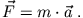U. Lechner, H.J. Ludde